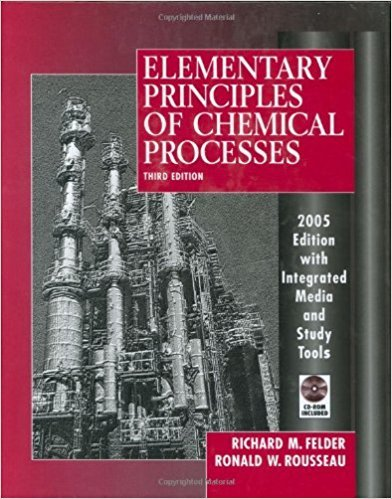×
Get Full Access to Elementary Principles Of Chemical Processes - 3 Edition - Chapter 4 - Problem 4.71
Get Full Access to Elementary Principles Of Chemical Processes - 3 Edition - Chapter 4 - Problem 4.71

×

# Liquid methanol is fed to a space heater at a rate of 12.0ISBN: 9780471687573 143

## Solution for problem 4.71 Chapter 4

Elementary Principles of Chemical Processes | 3rd Edition

• Textbook Solutions
• 2901 Step-by-step solutions solved by professors and subject experts
• Get 24/7 help from StudySoup virtual teaching assistantsElementary Principles of Chemical Processes | 3rd Edition

4 5 1 374 Reviews
30
4
Problem 4.71

Liquid methanol is fed to a space heater at a rate of 12.0 L/h and burned with excess air. The product gas is analyzed and the following dry-basis mole percentages are determined: CH30H = 0.45%, CO2 = 9.03%, and CO = 1.81 %. (a) Draw and label a flowchart and verify that the system has zero degrees of freedom. (b) Calculate the fractional conversion of methanol, the percentage excess air fed, and the mole fraction of water in the product gas. (c) Suppose the combustion products are released directly into a room. What potential problems do you see and what remedies can you suggest?

Step-by-Step Solution:

Problem 4.71

Liquid methanol is fed to a space heater at a rate of 12.0 L/h and burned with excess air. The product gas is analyzed and the following dry-basis mole percentages are determined: CH30H = 0.45%, CO2 = 9.03%, and CO = 1.81 %. (a) Draw and label a flowchart and verify that the system has zero degrees of freedom. (b) Calculate the fractional conversion of methanol, the percentage excess air fed, and the mole fraction of water in the product gas. (c) Suppose the combustion products are released directly into a room. What potential problems do you see and what remedies can you suggest?

Step by step solution

Step 1 of 3

a)

The equations for the complete and incomplete combustion of propane are given belowThe mole fractions of dry product gases are,Convert the volumetric flow rate (12.0L/h) to a molar flow rate, using its density (0.792 g/Ml) and molar mass (32.04 g/mol).Sketch and label the diagram for this process as shown below:Here,is molar flow rate of oxygen in the feed air, and n, is molar flow rate of water produced, x is the mole fraction of nitrogen in the dry product gas. The molar flow rate of nitrogen in the feed air is 3.76 (79/21) times the molar flow rate of oxygen in the feed air.

The mole fraction ofin the product gas is one minus the mole fractions of other dry gases.

The number of degrees of freedom is given by the equation.Here, unknowns is number of unknowns and depen, is number of independent equations that

can be written for the process.

In this process, the unknowns are molar flow ratesand mole fraction (x), so the number of unknowns is 4. The atomic balance equations can be written for C, H, O each and the mole balance for nitrogen molecule can be written.

So, the number of degrees of freedom is 0.= 4 - 4

= 0

Therefore, the number of degrees of freedom is 0.

_____________________________________________________________________________

Step 2 of 3

Step 3 of 3

##### ISBN: 9780471687573

The full step-by-step solution to problem: 4.71 from chapter: 4 was answered by , our top Chemistry solution expert on 11/15/17, 02:42PM. Elementary Principles of Chemical Processes was written by and is associated to the ISBN: 9780471687573. This textbook survival guide was created for the textbook: Elementary Principles of Chemical Processes, edition: 3. The answer to “Liquid methanol is fed to a space heater at a rate of 12.0 L/h and burned with excess air. The product gas is analyzed and the following dry-basis mole percentages are determined: CH30H = 0.45%, CO2 = 9.03%, and CO = 1.81 %. (a) Draw and label a flowchart and verify that the system has zero degrees of freedom. (b) Calculate the fractional conversion of methanol, the percentage excess air fed, and the mole fraction of water in the product gas. (c) Suppose the combustion products are released directly into a room. What potential problems do you see and what remedies can you suggest?” is broken down into a number of easy to follow steps, and 104 words. This full solution covers the following key subjects: air, Product, Mole, methanol, gas. This expansive textbook survival guide covers 13 chapters, and 710 solutions. Since the solution to 4.71 from 4 chapter was answered, more than 1303 students have viewed the full step-by-step answer.

Unlock Textbook Solution